## October 16th Foundation Exam

### Discrete Part I. Choose one question.

1. Prove by induction on n the following summation formula:Proof: We prove this identity by using induction on n ³ 1.

(Basis Step)

Consider n = 1. The summation on the LHS is:The formula on the RHS is: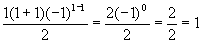Thus, LHS = RHS, so the Basis Step is proved.

(Induction Hypothesis)

Consider n = p. Assume the identity is true for n = p, for some p ³ 1; that is, assume(Induction Step)

Consider n = p + 1. We need to prove the identity is true for n = p + 1; that is, we need to prove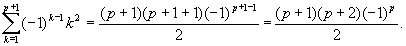Note that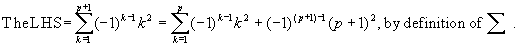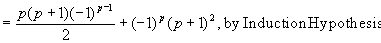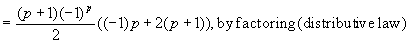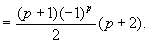So the LHS = RHS of the Induction Step.

Therefore, the identity of the problem is true for all n ³ 1.

2. Let g: A ® A be a bijective function. That is, g is both one-to-one and onto. For n ³ 2, define gn = g o g o Ö o g , where g is composed with itself n times. Prove that for n ³ 2, (1) gn is a bijective function from A to A; and (2) (gn)ñ1 = (gñ1)n.

Proof: We prove both (1) and (2) using induction on n ³ 2.

(Basis Step)

Consider n = 2. Since g: A ® A is a bijection, g2 = g o g is a bijection, by the theorem that says the compostion of two bijections is a bijection.

Also, (g2)ñ1 = (g o g)ñ1, by the definition of g2

= (gñ1) o (gñ1), by the theorem that says (h o f) ñ1= fñ1 o hñ1, when both f and h are bijections.

= (gñ1)2, by the definition of (gñ1)2.

Thus, the Basis Step is proved.

(Induction Hypothesis)

Consider n = k. Assume gk is a bijection, and (gk)ñ1 = (gñ1)k, for some k ³ 2.

(Induction Step)

Consider n = k + 1. We need to prove (1) gk +1 is a bijection; and (2) (gk +1)ñ1 = (gñ1)k +1.

Note that gk +1 = gk o g, by the definition of gk +1, so gk +1 is a bijection because gk is a bijection by the Induction Hypothesis, and its composition with g is also a bijection.

Further,

(gk +1)ñ1 = (gk o g)ñ1, by the definition of gk +1

= (gñ1) o (gk)ñ1, by the theorem (h o f) ñ1= fñ1 o hñ1

= (gñ1) o (gñ1)k, by the Induction Hypothesis

= (gñ1)k +1, by the definition of (gñ1)k +1.

Thus, the Induction Step is proved.

Therefore, we have proved that (1) gn is a bijective function from A to A; and (2) (gn)ñ1 = (gñ1)n, for all n ³ 2.

### Discrete Part II. Choose one question.

3. Let A = {a, b} denote a set of two symbols. Let S be a set of strings over A, satisfying the following property:

If u Î S and |u| > 0, then there exists a string v Î S such that u = avb.

Prove by induction that S Ì {anbn | n ³ 0}.

Proof: In order to prove S Ì {anbn | n ³ 0}, we need to prove that: if w Î S, then w Î {anbn | n ³ 0}.

We prove this statement by using induction on |w|, the length of string w.

(Basis Step)

Consider |w| = 0. In this case, w = l , the null string (you may use the symbol e.)

Since l = a0 b0, so w = l = a0 b0 Î {anbn | n ³ 0}. The Basis Step is proved.

(Induction Hypothesis)

Assume if w Î S, then w Î {anbn | n ³ 0} for all strings w with length |w| £ k, for some k ³ 0.

(Induction Step)

Consider the case if w Î S, and |w| = k + 1.

Note that |w| > 0, so there exists a string v Î S such that w = avb, by the property of S specified in the assumption. Since |v| = |w| - 2 = k + 1 - 2 = k - 1 £ k, so v Î {anbn | n ³ 0} by the Induction Hypothesis. That is,

v = ambm, for some m ³ 0.

Thus,

w = avb = a(ambm)b = am +1bm +1 Î {anbn | n ³ 0}.

So the Induction Step is proved.

Therefore, we have proved that

if w Î S, then w Î {anbn | n ³ 0} for strings w of any length |w| ³ 0.

That is, we have proved S Ì {anbn | n ³ 0}.

4. Let A = {a, b} denote a set of two symbols. Define the following two sets of strings over A:

C = {a}*{b}* , and

D = {w | w Î A*, and the number of occurrences of symbol a in w = the number of occurrences of symbol b in w}.

Prove that C Ç D = {anbn | n ³ 0}.

Proof: To prove C Ç D = {anbn | n ³ 0}, we prove

(1)   C Ç D Ì {anbn | n ³ 0}; and
(2)   {anbn | n ³ 0}Ì C Ç D, by the definition of set equality.

To prove (1), let string w Î C Ç D. Thus, w Î C and w Î D. Since {a}* = {an | n ³ 0} and {b}* = {bn | n ³ 0}, w Î C = {a}*{b}* implies w = ambp, for some m, p ³ 0. Also, since w Î D, m = p because the number of occurrences of symbol a in w must be equal to the number of occurrences of symbol b in w. Therefore, w = ambm Î {anbn | n ³ 0}, so (1) is proved.

To prove (2), let string w Î {anbn | n ³ 0}. Thus, w = ambm, for some m ³ 0. Therefore, w Î C ={a}*{b}*, because am Î {a}* and bm Î {b}*. Also, w Î D because the number of occurrences of symbol a in w = m = the number of occurrences of symbol b in w. Thus, w Î C Ç D, so (2) is proved.

Back to Oct 16 Foundation Exam page.